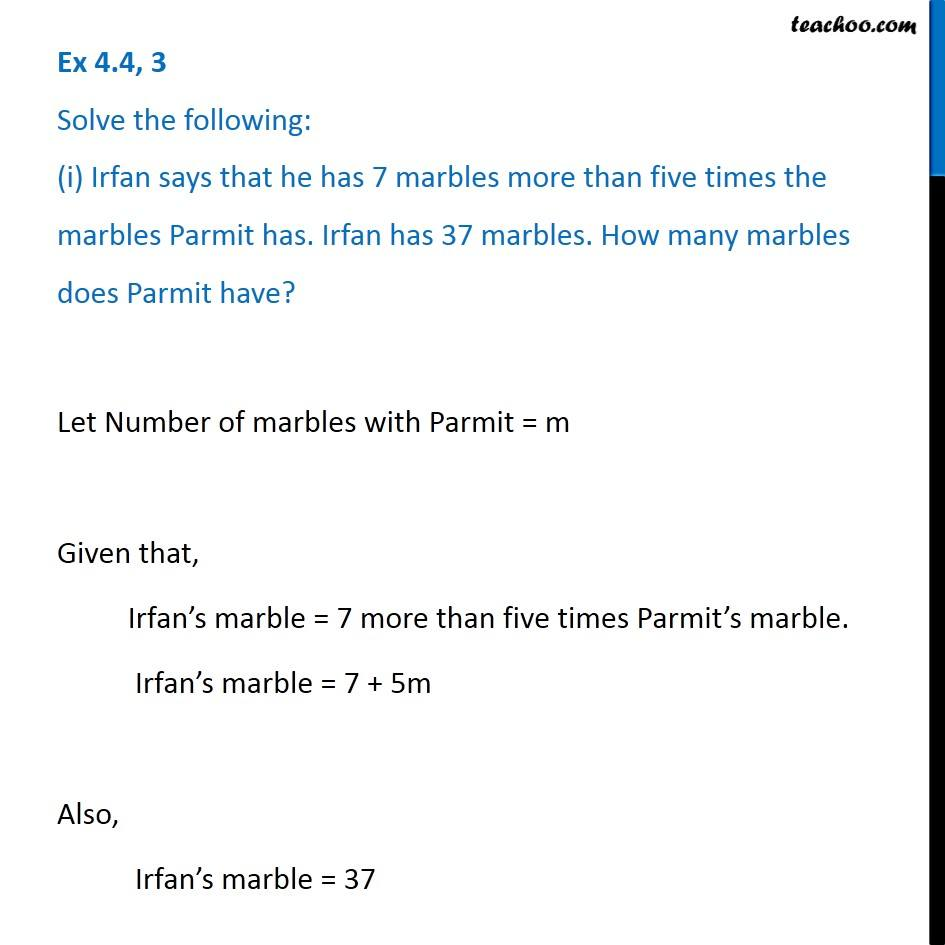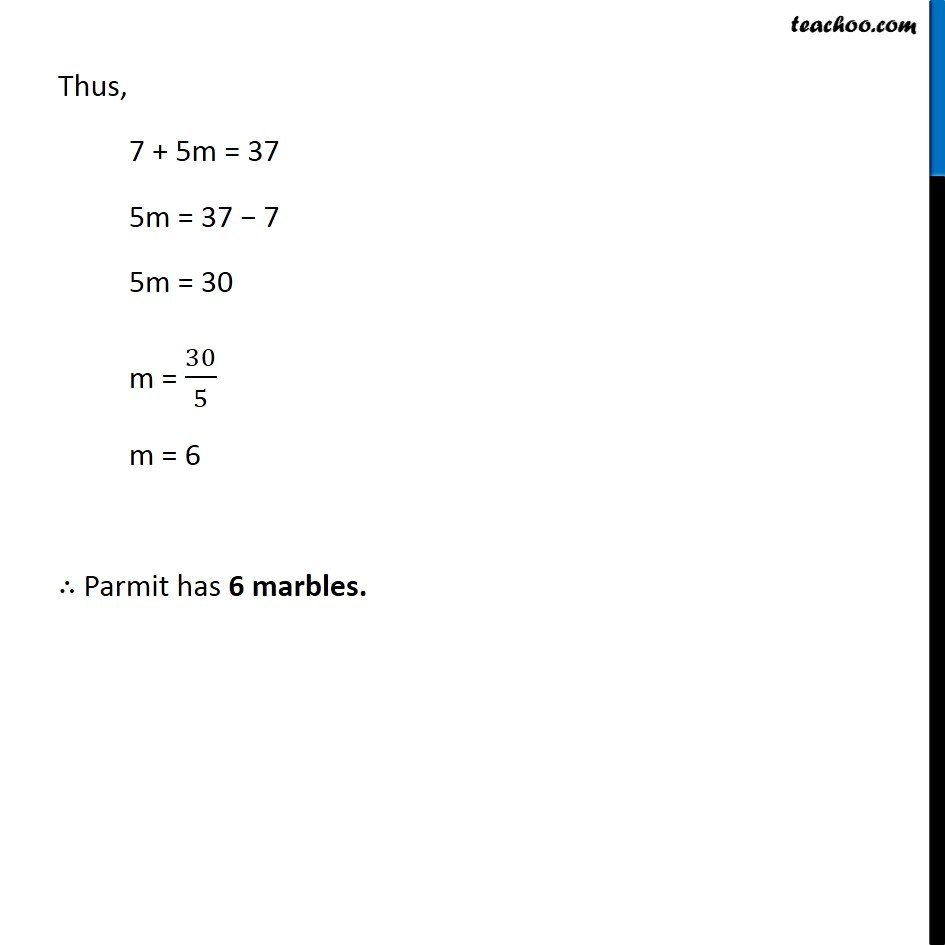Making equations from statements and solving

Chapter 4 Class 7 Simple Equations
Concept wiseIntroducing your new favourite teacher - Teachoo Black, at only ₹83 per month

### Transcript

Ex 4.4, 3 Solve the following: (i) Irfan says that he has 7 marbles more than five times the marbles Parmit has. Irfan has 37 marbles. How many marbles does Parmit have?Let Number of marbles with Parmit = m Given that, Irfan’s marble = 7 more than five times Parmit’s marble. Irfan’s marble = 7 + 5m Also, Irfan’s marble = 37 Thus, 7 + 5m = 37 5m = 37 − 7 5m = 30 m = 30/5 m = 6 ∴ Parmit has 6 marbles.